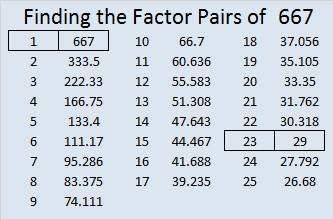# 667 and Level 2

Since 29 is one of its factor, 667 is the hypotenuse of the Pythagorean triple 460-483-667. What is the greatest common factor of those three numbers?

The numbers in the factor pair 23 and 29 are each exactly three numbers away from 26, their average. That means we are just 3² numbers away from 26².

Indeed, 667 equals 26² – 3² which can be factored into (26 + 3)(26 – 3) so (26 + 3)(26 – 3) = 29 x 23 = 667.Print the puzzles or type the solution on this excel file: 12 Factors 2015-11-02

—————————————————————————————————

• 667 is a composite number.
• Prime factorization: 667 = 23 x 29
• The exponents in the prime factorization are 1 and 1. Adding one to each and multiplying we get (1 + 1)(1 + 1) = 2 x 2 = 4. Therefore 667 has exactly 4 factors.
• Factors of 667: 1, 23, 29, 667
• Factor pairs: 667 = 1 x 667 or 23 x 29
• 667 has no square factors that allow its square root to be simplified. √667 ≈ 25.826343.—————————————————————————————————